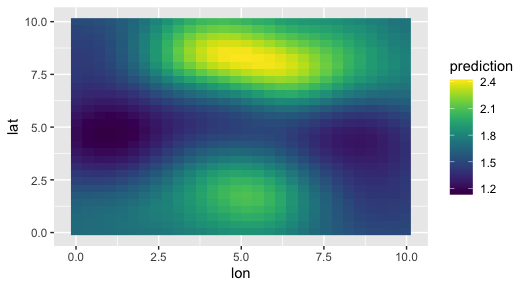# Spatial GLMs with glmmfields

#### 2023-03-10

Here we will use the glmmfields package to fit a spatial GLM with a predictor. While glmmfields was designed to fit spatiotemporal GLMs with the possibility of extreme events, it can also be used to fit regular spatial GLMs without a time element and without extreme events. Currently it can fit Gaussian (link = identity), Gamma (link = log), Poisson (link = log), negative binomial (link = log), and binomial (link = logit) models. The package can also fit lognormal (link = log) models.

library(glmmfields)
library(ggplot2)
library(dplyr)

Set up parallel processing (not used in this example):

options(mc.cores = parallel::detectCores())

First, let’s simulate some data. We will use the built-in function sim_glmmfields(), but normally you would start with your own data. We will simulate 200 data points, some (fake) temperature data, an underlying random field spatial pattern, and add some observation error. In this example we will fit a Gamma GLM with a log link.

The underlying intercept is 0.5 and the slope between temperature and our observed variable (say biomass or density of individuals in a quadrat) is 0.2.

set.seed(1)
N <- 200 # number of data points
temperature <- rnorm(N, 0, 1) # simulated temperature data
X <- cbind(1, temperature) # our design matrix
s <- sim_glmmfields(
n_draws = 1, gp_theta = 1.5, n_data_points = N,
gp_sigma = 0.2, sd_obs = 0.2, n_knots = 12, obs_error = "gamma",
covariance = "squared-exponential", X = X,
B = c(0.5, 0.2) # B represents our intercept and slope
)
d <- s$dat d$temperature <- temperature
ggplot(s$dat, aes(lon, lat, colour = y)) + viridis::scale_colour_viridis() + geom_point(size = 3)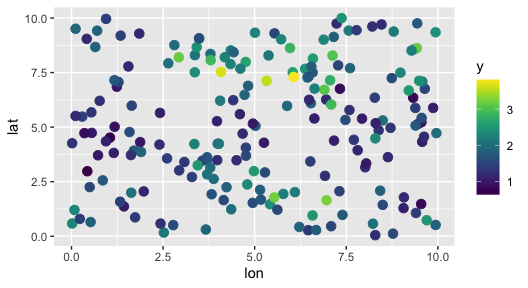If we fit a regular GLM we can see that there is spatial autocorrelation in the residuals: m_glm <- glm(y ~ temperature, data = d, family = Gamma(link = "log")) m_glm ## ## Call: glm(formula = y ~ temperature, family = Gamma(link = "log"), ## data = d) ## ## Coefficients: ## (Intercept) temperature ## 0.5369 0.1967 ## ## Degrees of Freedom: 199 Total (i.e. Null); 198 Residual ## Null Deviance: 23.27 ## Residual Deviance: 16.72 AIC: 280.9 confint(m_glm) ## 2.5 % 97.5 % ## (Intercept) 0.4964271 0.5780115 ## temperature 0.1522553 0.2413277 d$m_glm_residuals <- residuals(m_glm)
ggplot(d, aes(lon, lat, colour = m_glm_residuals)) +
geom_point(size = 3)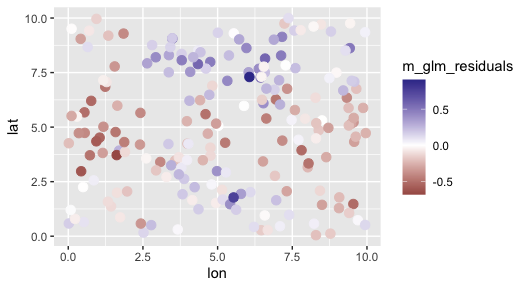Let’s instead fit a spatial GLM with random fields. Note that we are only using 1 chain and 500 iterations here so the vignette builds quickly on CRAN. For final inference, you should likely use 4 or more chains and 2000 or more iterations.

m_spatial <- glmmfields(y ~ temperature,
data = d, family = Gamma(link = "log"),
lat = "lat", lon = "lon", nknots = 12, iter = 500, chains = 1,
prior_intercept = student_t(3, 0, 10),
prior_beta = student_t(3, 0, 3),
prior_sigma = half_t(3, 0, 3),
prior_gp_theta = half_t(3, 0, 10),
prior_gp_sigma = half_t(3, 0, 3),
seed = 123 # passed to rstan::sampling()
)
## Warning: Bulk Effective Samples Size (ESS) is too low, indicating posterior means and medians may be unreliable.
## Running the chains for more iterations may help. See
## https://mc-stan.org/misc/warnings.html#bulk-ess
## Warning: Tail Effective Samples Size (ESS) is too low, indicating posterior variances and tail quantiles may be unreliable.
## Running the chains for more iterations may help. See
## https://mc-stan.org/misc/warnings.html#tail-ess

Let’s look at the model output:

m_spatial
## Inference for Stan model: glmmfields.
## 1 chains, each with iter=500; warmup=250; thin=1;
## post-warmup draws per chain=250, total post-warmup draws=250.
##
##            mean se_mean   sd   2.5%    25%    50%    75%  97.5% n_eff Rhat
## gp_sigma   0.27    0.00 0.06   0.17   0.22   0.26   0.31   0.40   256 1.00
## gp_theta   1.62    0.01 0.19   1.27   1.49   1.60   1.75   2.04   226 1.00
## B       0.49    0.01 0.06   0.36   0.45   0.49   0.54   0.61    22 1.04
## B       0.19    0.00 0.02   0.16   0.18   0.19   0.20   0.22   329 1.00
## CV      0.21    0.00 0.01   0.19   0.20   0.21   0.22   0.23   253 1.00
## lp__     -62.61    0.29 3.00 -70.08 -64.18 -62.31 -60.45 -57.78   109 1.00
##
## Samples were drawn using NUTS(diag_e) at Fri Mar 10 14:07:11 2023.
## For each parameter, n_eff is a crude measure of effective sample size,
## and Rhat is the potential scale reduction factor on split chains (at
## convergence, Rhat=1).

We can see that the 95% credible intervals are considerably wider on the intercept term and narrower on the slope coefficient in the spatial GLM vs. the model that ignored the spatial autocorrelation.

Let’s look at the residuals in space this time:

plot(m_spatial, type = "spatial-residual", link = TRUE) +
geom_point(size = 3)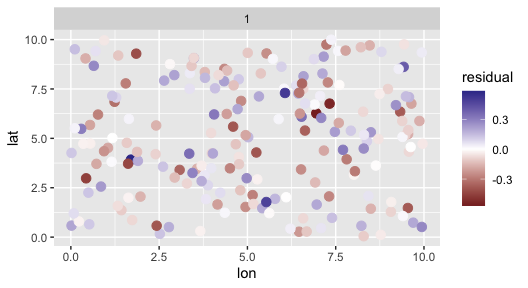That looks better.

We can inspect the residuals versus fitted values:

plot(m_spatial, type = "residual-vs-fitted")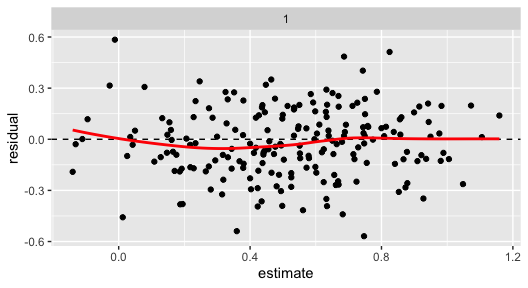And the predictions from our model itself:

plot(m_spatial, type = "prediction", link = FALSE) +
viridis::scale_colour_viridis() +
geom_point(size = 3)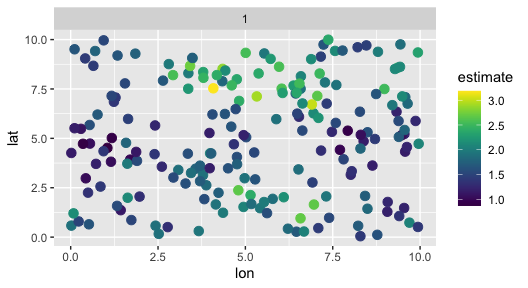Compare that to our data at the top. Note that the original data also includes observation error with a CV of 0.2.

We can also extract the predictions themselves with credible intervals:

# link scale:
p <- predict(m_spatial)
head(p)
## # A tibble: 6 × 3
##   estimate conf_low conf_high
##      <dbl>    <dbl>     <dbl>
## 1    0.726   0.639      0.822
## 2    0.561   0.450      0.664
## 3    0.187   0.0567     0.332
## 4    0.922   0.788      1.06
## 5    0.624   0.509      0.726
## 6    0.494   0.380      0.628
# response scale:
p <- predict(m_spatial, type = "response")
head(p)
## # A tibble: 6 × 3
##   estimate conf_low conf_high
##      <dbl>    <dbl>     <dbl>
## 1     2.07     1.89      2.28
## 2     1.75     1.57      1.94
## 3     1.21     1.06      1.39
## 4     2.51     2.20      2.89
## 5     1.87     1.66      2.07
## 6     1.64     1.46      1.87
# prediction intervals on new observations (include observation error):
p <- predict(m_spatial, type = "response", interval = "prediction")
head(p)
## # A tibble: 6 × 3
##   estimate conf_low conf_high
##      <dbl>    <dbl>     <dbl>
## 1     2.07    1.39       2.99
## 2     1.75    1.09       2.59
## 3     1.21    0.747      1.82
## 4     2.51    1.54       3.81
## 5     1.87    1.15       2.67
## 6     1.64    0.996      2.39

Or use the tidy method to get our parameter estimates as a data frame:

head(tidy(m_spatial, conf.int = TRUE, conf.method = "HPDinterval"))
## # A tibble: 6 × 5
##   term                     estimate std.error conf.low conf.high
##   <chr>                       <dbl>     <dbl>    <dbl>     <dbl>
## 1 gp_sigma                    0.256    0.0597    0.167     0.372
## 2 gp_theta                    1.60     0.192     1.25      1.98
## 3 B                        0.492    0.0619    0.370     0.615
## 4 B                        0.190    0.0159    0.162     0.226
## 5 CV                       0.209    0.0109    0.190     0.230
## 6 spatialEffectsKnots[1,1]    0.319    0.0785    0.186     0.491

Or make the predictions on a fine-scale spatial grid for a constant value of the predictor:

pred_grid <- expand.grid(
lat = seq(min(d$lat), max(d$lat), length.out = 30),
lon = seq(min(d$lon), max(d$lon), length.out = 30)
)
pred_grid$temperature <- mean(d$temperature)
pred_grid$prediction <- predict( m_spatial, newdata = pred_grid, type = "response" )$estimate
ggplot(pred_grid, aes(lon, lat, fill = prediction)) +
geom_raster() +
viridis::scale_fill_viridis()